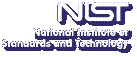UpApproximating Large Frequency Moments with $O(n^{1−2/k})$ Bits

Department of Computer Science, Johns Hopkins University

Tuesday, February 24, 2015 15:00-16:00,
Building 101, Heritage Room
Gaithersburg
Tuesday, February 24, 2015 13:00-14:00,
1-4058
Boulder

Abstract:

We consider the problem of approximating frequency moments in the streaming model. Given a stream $D = \{p_1,p_2,...,p_m\}$ of numbers from $\{1,...,n\}$, a frequency of $i$ is defined as $f_i = |{j: p_j = i}|$. The $k$-th frequency moment of D is defined as $F_k = \sum_{i=1}^n f_i^k$.

In their celebrated paper, Alon, Matias, and Szegedy (STOC 1996) introduced the problem of computing a $(1 \pm \epsilon)$-approximation of $F_k$ with sublinear memory. We give upper bound of $O(n^{1-2/k})$ bits that matches, up to a constant factor, the lower bound of Woodruff and Zhang (STOC 12) for constant $\epsilon$ and $k > 3$.

Joint work with Jonathan Katzman, Charles Seidell and Gregory Vorsanger.

Contact: J. Shook

Note: Visitors from outside NIST must contact Cathy Graham; (301) 975-3800; at least 24 hours in advance.